Microsoft Excel quick and easy

New Excel tutorial every week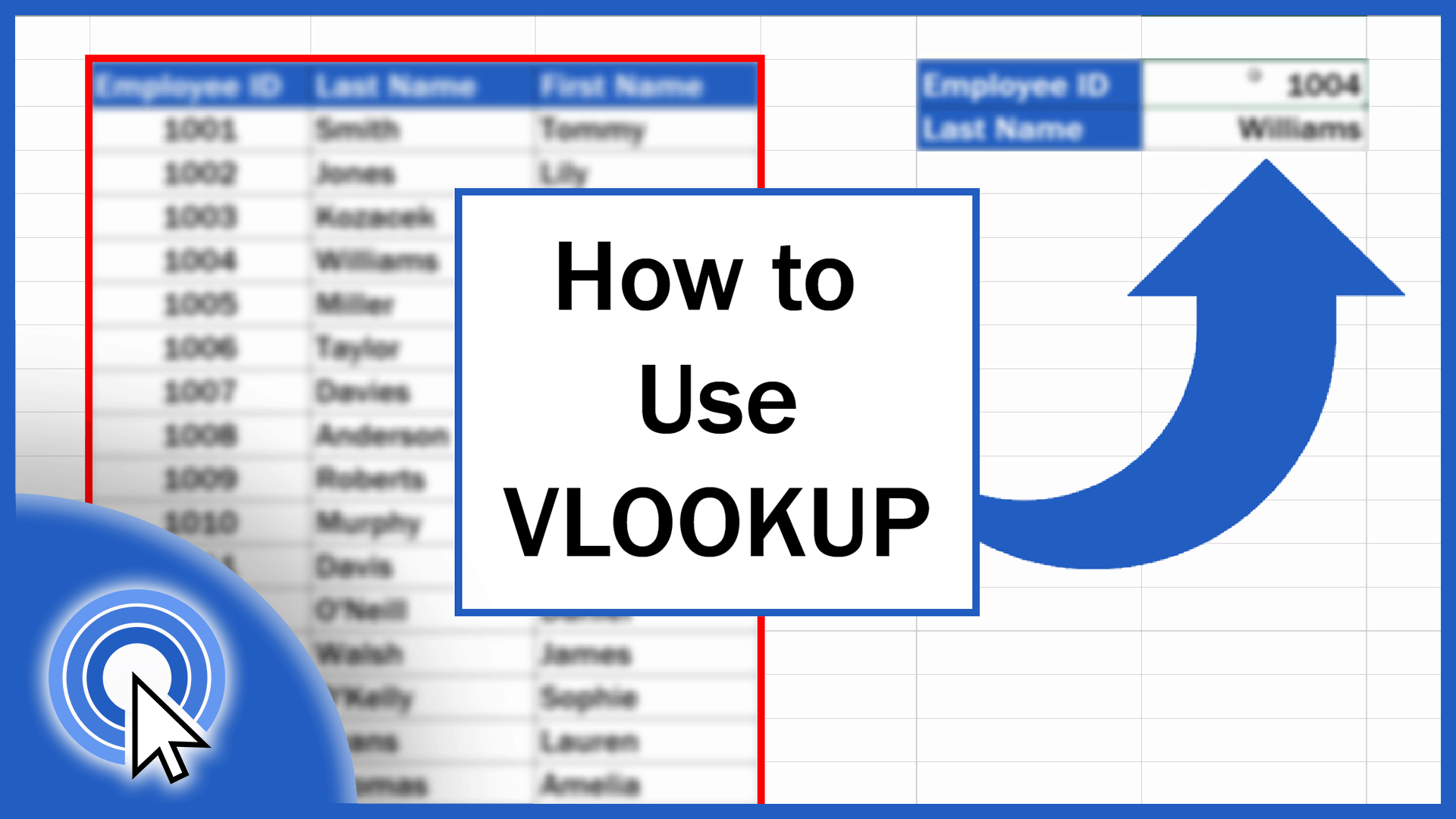## How to Use the VLOOKUP Function in Excel (Step by Step)

This tutorial offers a detailed description of how to use VLOOKUP in Excel. The VLOOKUP function in Excel performs a case-insensitive lookup.## How to Calculate the Range in Excel (in 3 easy steps)

Learn three easy steps to calculate the range of a set of data in Excel, no matter how many values there are in the data table you’re working with.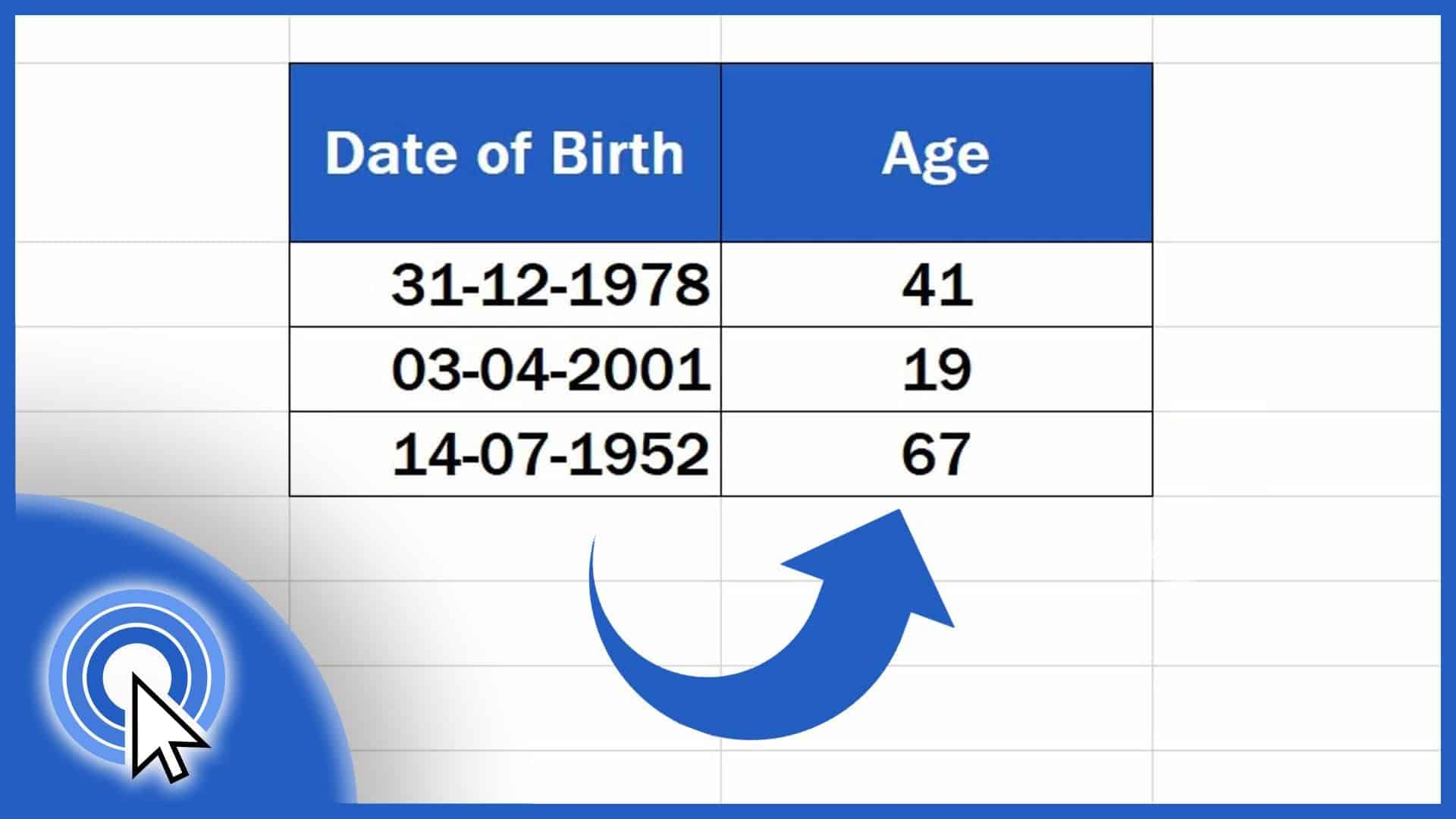## How to Calculate Age Using a Date of Birth in Excel (The Easy Way)

Learn how to calculate age using a date of birth in Excel. You’ll be able to find out the exact number of years that passed from a date of birth until now.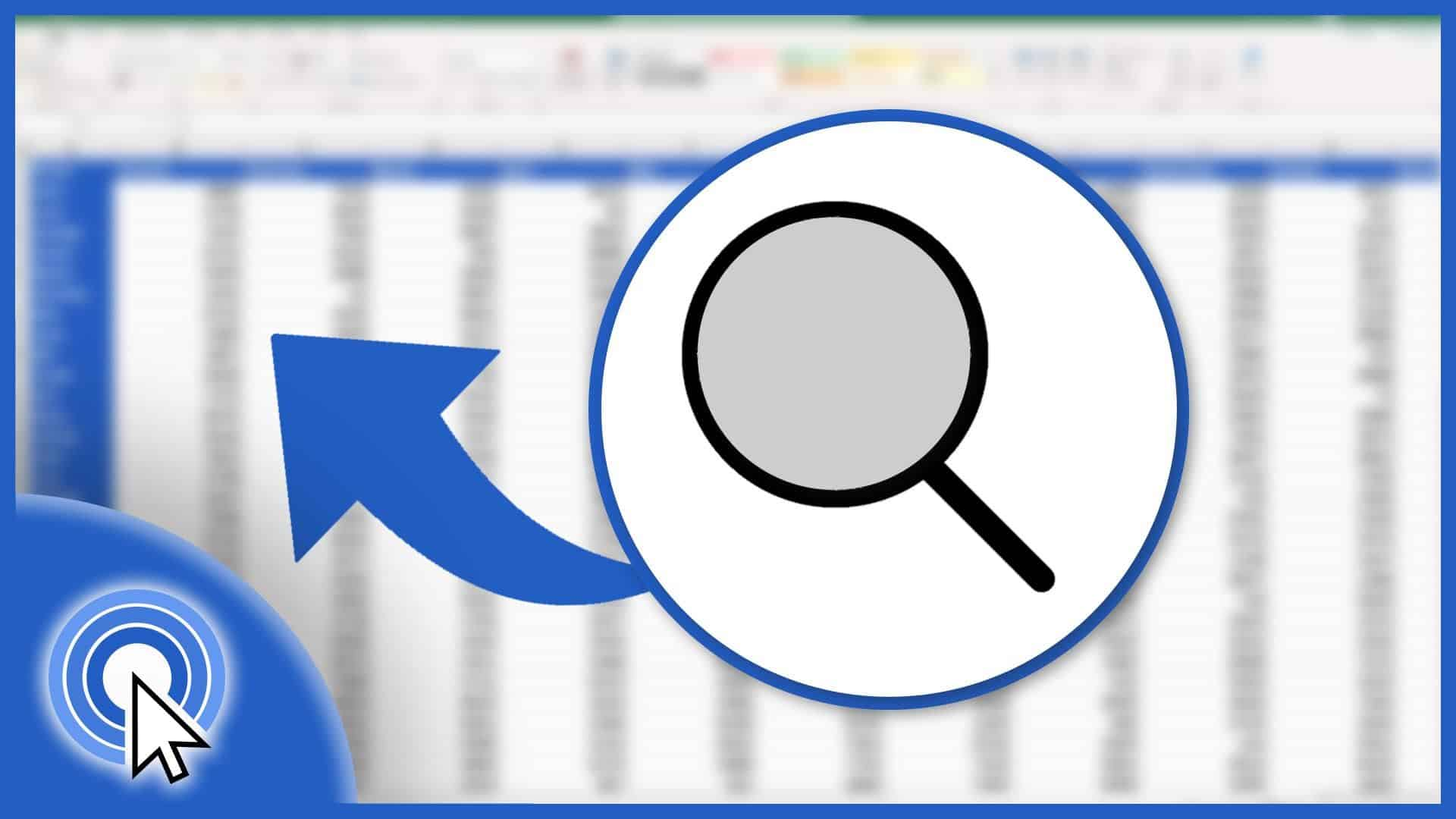## How to Search in Excel Sheet (From Basic to Advanced)

Sometimes the function Search in Excel comes handy. Let’s have a look together at how to search in an Excel sheet in a simple and easy way.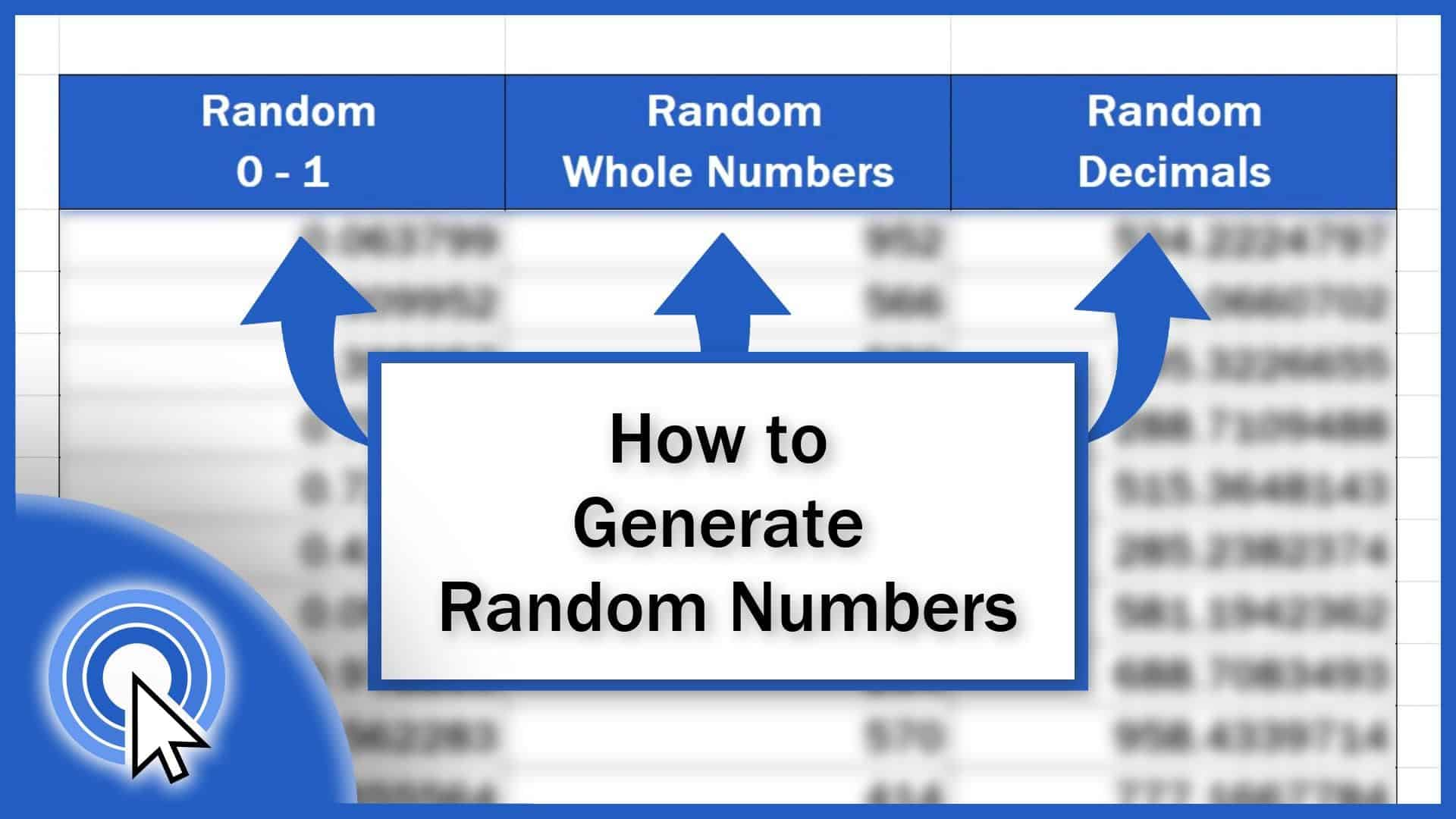## How to Generate Random Numbers in Excel (3 Different Ways)

This tutorial covers how to generate random numbers within any range you define, whether you need to generate whole numbers or decimals.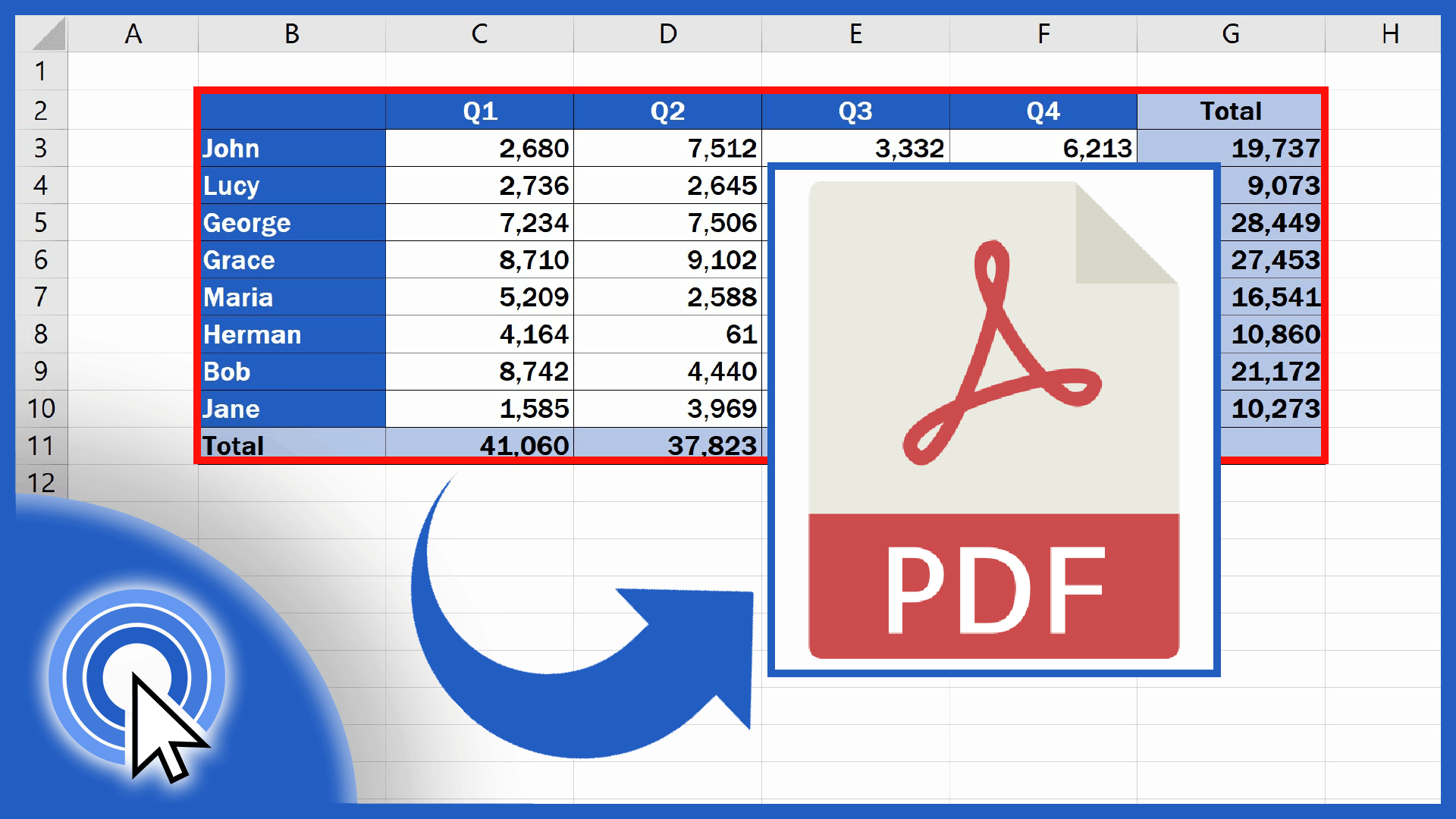## How to Convert an Excel File into PDF

This tutorial is about how to convert an Excel file into PDF conveniently, directly in Excel, so that you wouldn’t need to install any new software.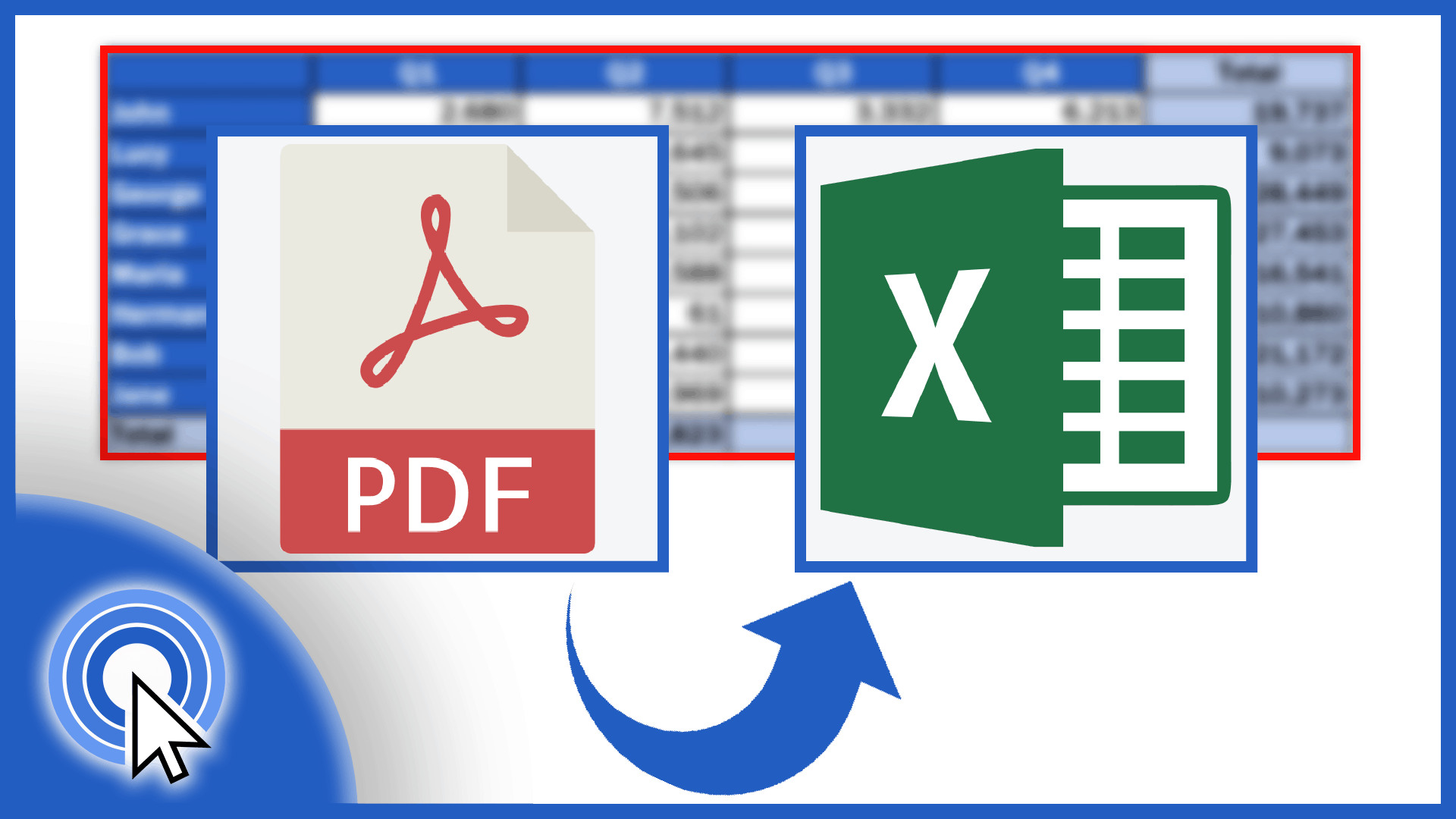## How to Convert PDF to an Excel File (Quick and Easy)

Learn how to convert PDF to an Excel file. In this tutorial, we’re gonna have a look at how to manage all this, simple and easy.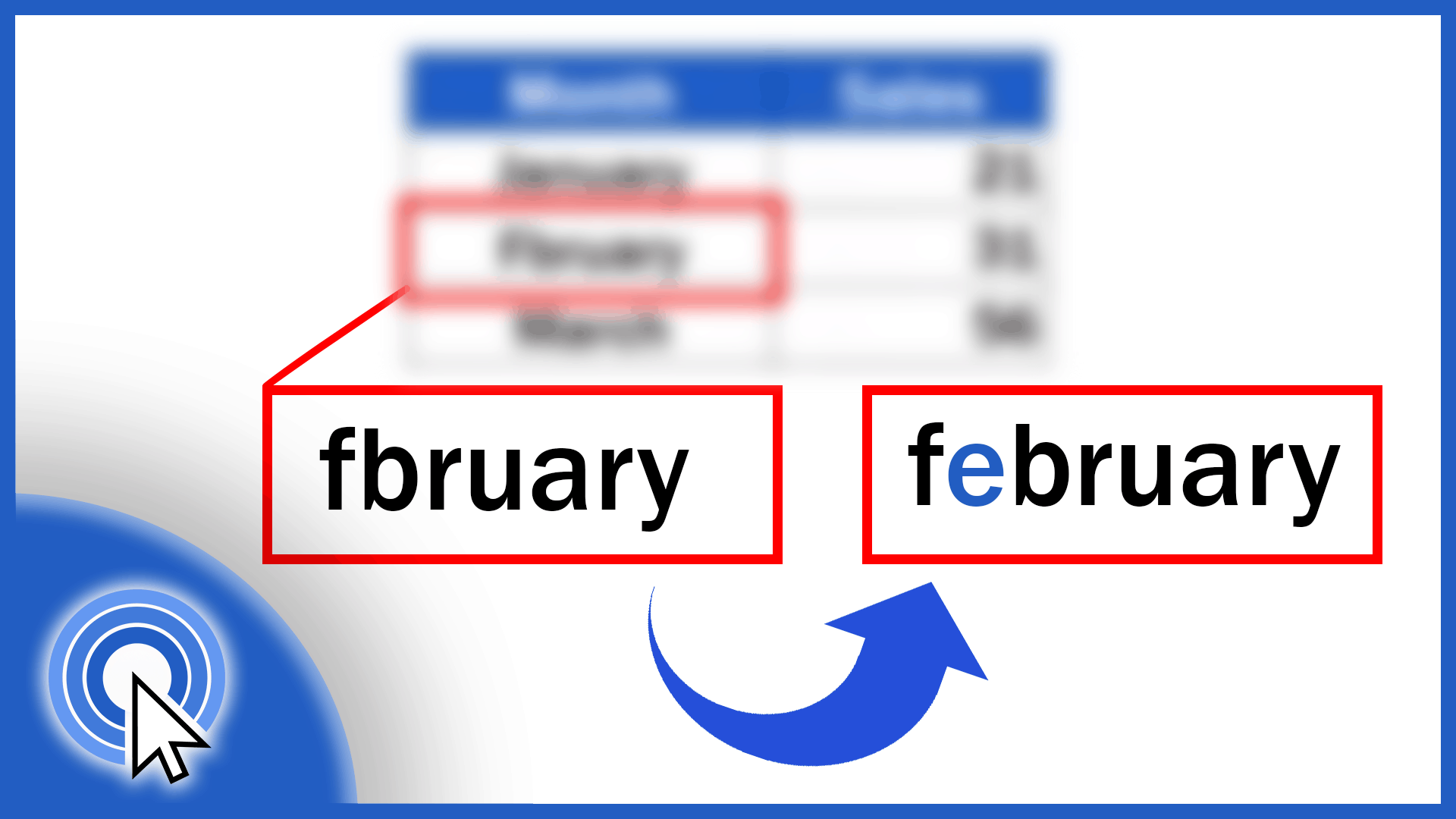## How to Check Spelling in Excel and Avoid Typos

Learn how to use spellcheck function in Excel. It is an invaluable help when it comes to avoiding typos or grammatical issues in texts within data tables.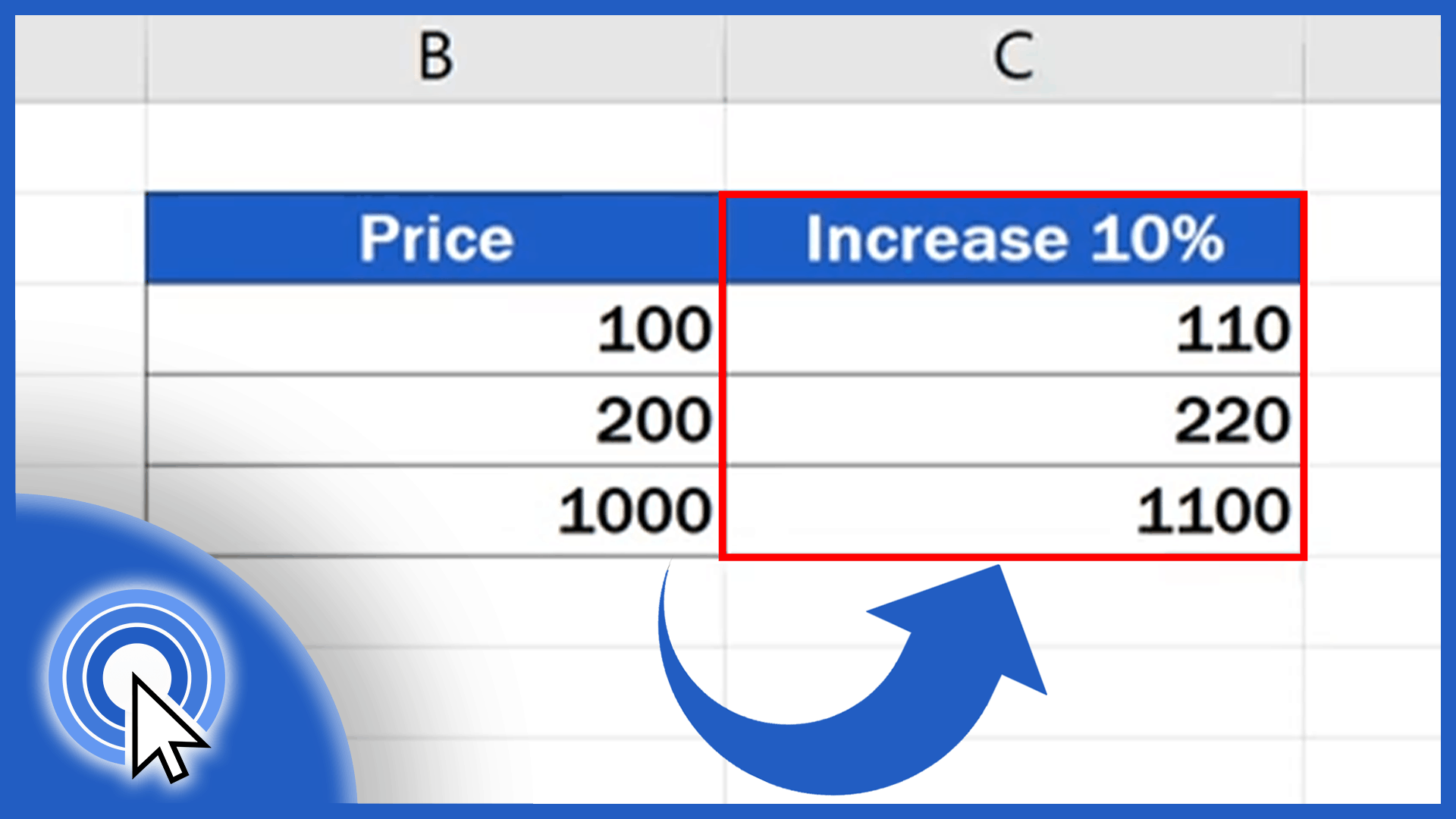## How to Calculate Percentage Increase in Excel (The Right Way)

Learn three different ways how to calculate percentage increase in Excel. All of them are correct and can be used interchangeably to get the same result.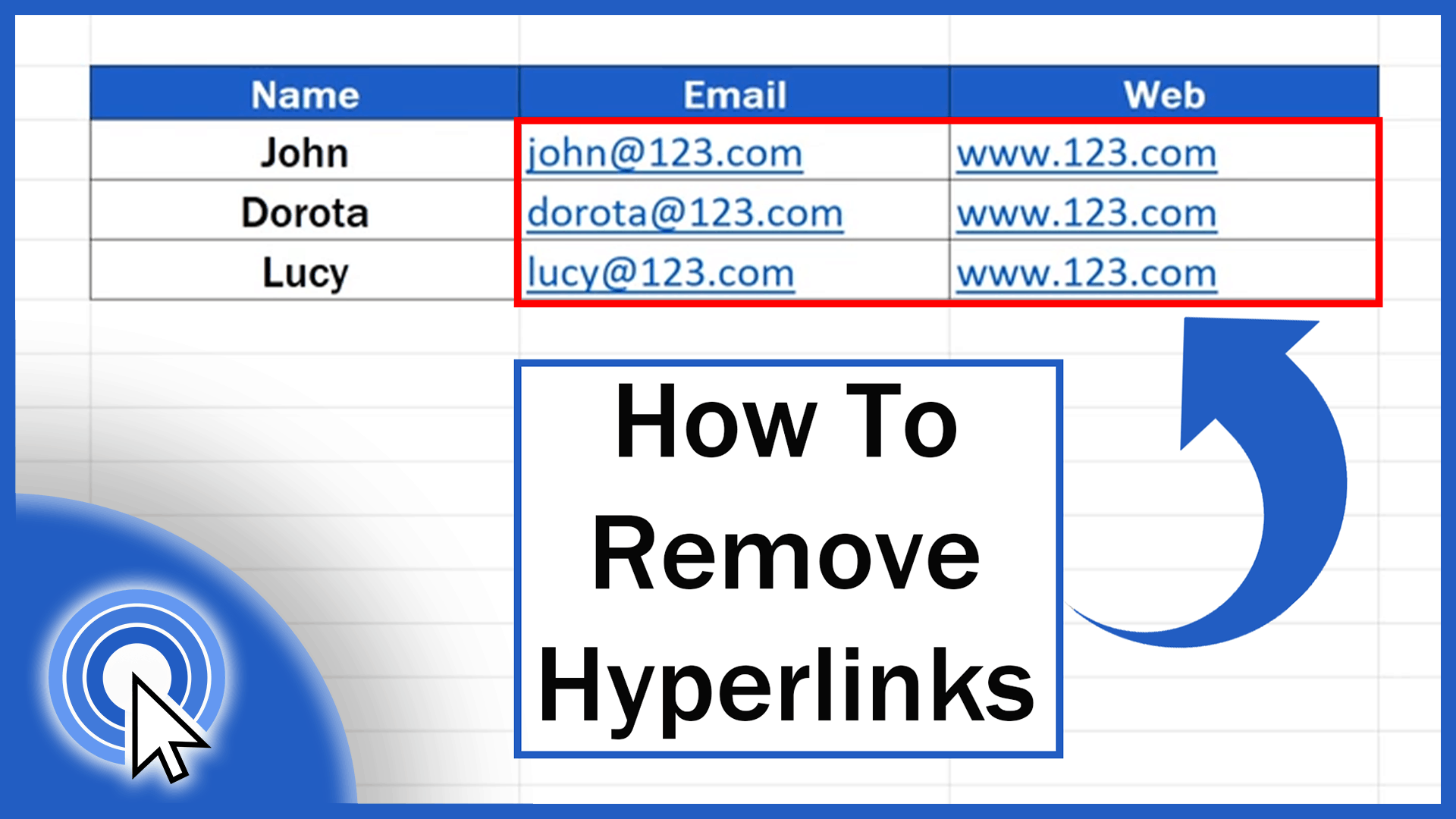## How to Remove Hyperlinks in Excel

Excel will automatically insert a hyperlink when you enter a website or an email address into a cell. Learn how to remove hyperlinks in Excel easily.